# 2.2: Weak Acids and Bases, pH and pKa

The earlier section described the general acid/base properties of water. There are many functional groups in both small and large biomolecules that act as acids and bases. Common weak acids are carboxylic acids and derivatives of phosphoric acid which become negatively charge on donation of a proton. Common weak base are amines which become positively charged on protonation. Such charge acquisition changes the properties of the acid or base. A protonated amine is no longer a nucleophile. A deprotonated carboxylic acid can now engaged in an ion-ion IMF.

The extent of deprotonation depends on the acidity/basicity of the environment. We have to turn to a bit of mathematics to determine that extent.

### Reaction of water with self: autoionization

As shown in the previous section, water can react with itself to produce H3O+ and OH-.This reaction is often represented in a simpler form:

H2O ↔ H+ + OH-.

The equilibrium constant for this simplified reaction can be written as

$K_{eq}=\dfrac{[H^{+}][OH^{-}]}{H_{2}O}$

Given the know value of Keq and the concentration of water (55 M), this can be rewritten as

$K_a=55 K_{eq} = [H^{+}][OH^{-}] = 10^{-14}$

Hence pure, neutral water has equal but small concentrations, 10-7 M of H3O+ and OH-.

You remember from introductory chemistry and life in general that the pH of pure water is 7. This derives from the general formulas for both pH and a new quantity, pKa.

$pH = -log[H_3O^+] = -log(10^{-7}) = 7$

$pK_a = -logK_a = -log(10^{-14}) = 14$

(Note: some books use 15.7 for the pKa of water. Here is a link to an explanation of why 14 is better.)

All acids of the generic formula HA have pKa.

HA ↔ H+ + A-

The equilibrium constant for this simplified reaction can be written as

$K_{eq}=\dfrac{[H^{+}][A^{-}]}{HA}$

$K_a=[HA] K_{eq} = [H^{+}][A^{-}]$

$pK_a = -logK_a$

The pKa becomes a simple measure of the strength of an acid. The stronger the acid, the larger the Ka and the smaller the pKa.

Here is table of pKa values for common acids and functional groups. The pKa values change with different substituents on the acids differ. The stronger the acid, the weaker the conjugate base. This should make sense as a weak base is unlikely to reabstract a proton and return to its original acidic form. Likewise, the weakest acids produce the strongest conjugate bases which reprotonate to return to the weak acid state.

 Group Example weaker acid ≈ pKa Conjugate Base stronger conj. base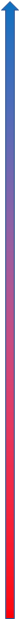alkane50amine35alkyne25alcohol16water14protonated amine10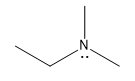phenol10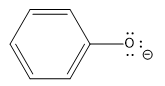thiol10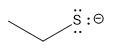imidazole7carboxylic acid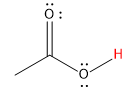5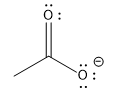hydrochloric acid-8stronger acid weaker conj. base

### The Henderson-Hasselbalch Equation

We can find the pKa for small acids in solution in pKa tables. However, from a biochemical perspective, we often need to know the charge state of the acid. Since the pH is approximately constant in organisms (more on that later), we know the [H3O+ ]. Hence we can calculate the ratio of $$A^- / HA$$ using the Henderson-Hasselbalch equation, which is derived below.

HA ↔ H+ + A-

$K_a=\dfrac{[H^{+}][A^{-}]}{HA}$

$-log K_a = - log [H^{+}] - log ([A^{-}]/[HA])$

$pK_a = pH - log ([A^{-}]/[HA])$

$pH = pK_a + log \dfrac{[A^{-}]}{HA}$

In your chemistry class you certainly would have performed titration curve analyses acids. What is the chemistry that occurs at each step. Let's assume the pH is low and much lower than the pKa of the acid. From the Henderson-Hasselbalch equation, you would surmise that the ratio of A-/HA is very small - that is the acid is essentially fully protonated. The should also make intutitive sense. For an weak acid to be coaxed to give up a proton, a reasonably strong base (like OH-) should be added. So at low pH the acids exists just as HA. Now consider adding an amount to NaOH to match the concentration of the ionizable proton. At that point in the titration, mass balance would suggest that the acid in its protonated state is gone, and all that remains is A-. What happens if just enough NaOH is added to react with half of the HA. The mass balance would tell us that A-=HA and at that point, the pH = pKa of the acid.

The entire titration curve can be calculated from the Henderson-Hasselbalch equation. A graph of it is shown below.

The graph simply shifts up as the pKa is increased. The pH starts soaring at the end of the graph after the added hydroxide has reacted with the last ionizable proton. After that the pH is determined by the concentration of the strong base OH-.

The graph is flattest in the middle of curve at the inflection point of the curve. Note at this pH, pH = pKa. In the middle of the curve, the pH changes least on addition of small amounts of OH-. This is the basis of buffering which will be covered in the next section.

IF you know the pH of a solution and the pKa of the ionizable group, you can very quickly estimate the average charge state of the function group. Here is the Henderson-Hasselbach equation again:

$pH = pK_a + log \dfrac{[A^{-}]}{HA}$

Let's see what happen under 3 specifi pH states:

1. If the pH is 2 units below the pKa (i.e under more acidic condition when you would expect the group to be protonated), the equation becomes,$$-2 = log A/HA, or .01 = A/HA$$. This means that the functional group will be about 99% protonated (with either 0 or +1 charge, depending of the functional group).
2. If the pH is 2 units above the pKa, the equation becomes $$2 = log A/HA, or 100 = A/HA$$. Therefore the functional group will be 99% deprotonated.
3. If the pH = pka, the HH equation becomes $$0 = log A/HA, or 1 = A/HA$$. Therefore the functional group will be 50% deprotonated

From these simple examples, we have illustrate the +2 rule to determine charge state. This rule is used to quickly determine protonation, and hence charge state, and is extremely important to know (and easy to derive).

### Polyprotic Oxyacids

Acids that can donate more than one proton are called polyprotic acids. They are typically oxyacids, with the ionizable proton on an oxygen atom, which can form a reasonably stable oxyanion (negative on the oxygen) as the oxygen is electronegative and stabilize the charge. The negative charge on the conjugate base of oxyacids is further stabilized by resonance delocalization involving the doubly bonded oxygen atom. Two of the most biologically relevant oxyacids are shown in the figure below.The pKa for each subsequent ionization is higher since it is more difficult to remove a proton from an increasingly more charged molecular ion. The titration plot of pH vs NaOH is similar to the graph above but has multiple plateaus at pH=pKa,

Derivatives of phosphoric acid are found in all major classes of biomolecules. Nucleic acid contain a sugar-phosphate link in their backbone. Many proteins become phosphorylated after they are synthesized. Structure lipids contain a phosphate in their. Like whole classes of phospholipids are found in biomembranes.

### Charge State of Biomolecules

The Henderson-Hasselbalch equation can be used to determined the charge state of ionizable functional groups (carboxylic and phosphoric acids, amines, imidazoles, guanidino groups) even on a large macromolecule such as proteins and DNA. Large proteins contain carboxylic acid groups.

The figure below shows how the weakly acidic aspartic and glutamic acids, two common amino acids found in proteins, contribute negative charge to the protein.Other amino acids that contain an alcoholic function group can also become phosphorylated to produce a phosphoprotein, which converts a neutral ROH group to a phosphoester with a negative two charge.### pKa and Environment

The pKa is really a measure of the equilibrium constant for the reaction. And of course, you remember that ΔGo = -RT ln Keq. Therefore, pKa is independent of concentration, and depends only on the intrinsic stability of reactants with respect to the products.  However, this is true only under a given set of conditions such as temperature, pressure and solvent composition.

Consider, for example acetic acid, which in aqueous solution has a pKa of about 4.7. It is a weak acid, which dissociates only slightly to form H+ (in water the hydronium ion, H3O+, is formed) and acetate (Ac-). These ions are moderately stable in water, but reassociate readily to form the starting product. The pKa of acetic acid in 80% ethanol is 6.87. This can be accounted for by the decrease in stability of the charged products, which are less shielded from each other by the less polar ethanol. Ethanol has a lower dielectric constant than does water. The pKa increases to 10.32 in 100% ethanol, and to a whopping 130 in air!

The pKa values of ionizable groups in proteins vary enormously as they depend on the microenvironment of the group.  Consider the amino acid asparatic acid (Asp, D), which has a -CH2CO2H  R-group or "side chain" similar to acetic acid.  In a given protein, a given Asp side chain might be on the surface but another in the same protein might be buried in the protein away from water.  You would expect the pKa values for these two different Asp side chains to be different.  The average pKa for Asp side chains in 78 different proteins was shown to be 3.5, less than that of acetic acid (4.7) but not dramatically.  However the range of pKa values for Asp in these proteins was huge, with the lowest being 0.5 (a buried Asp in the protein T4 Lysosome) and the highest being 9.2 in the protein thioredoxin from E. Coli.

The iCn3D model below shows the surrounding environment of Asp 70 (D70) in T4 Lysoszyme.  Its pKa has been determined experimentally to be 0.5,way stronger than acetic acid .  The dotted cyan lines show ion - ion interactions between the -CH2CO2- side chain of Asp 70 (D7) and the positively charge imidazolium group of histidine (H31) in the protein.  The distance between the two charged groups is about 3.4 A (see second set of icn)

https://structure.ncbi.nlm.nih.gov/i...n8JvA6q2E8U857

add second icn to see distance

https://structure.ncbi.nlm.nih.gov/i...E7vdxL6pCX2jy5

https://www.ncbi.nlm.nih.gov/Structu...2&command=view annotations; set annotation cdd; hide annotation cdd; set view detailed view; select .A:70 | name Asp70; select zone cutoff 5 | sets selected non-selected | false; select .A:30-31 or .A:66-69 or .A:71-74 or .A:104 or .Misc:1 or .Misc:9 or .Misc:23 or .Misc:70 or .Misc:79 or .Misc:110 or .Misc:117 | name 5A_Asp70; select .A:30-31 or .A:66-74 or .A:104 or .Misc:1 or .Misc:9 or .Misc:23 or .Misc:70 or .Misc:79 or .Misc:110 or .Misc:117 | name Asp70with5A; show selection; style proteins stick; color atom; set background white; add residue number labels; set labels off; select sets Asp70; add residue number labels; pickatom 257; add residue number labels; select sets Asp70with5A; display interaction 3d | selected selected | salt bridge | false | threshold 3.8 6 4 3.8 6 5.5; set annotation cdd; pickatom 550; add line | x1 35.37 y1 15.33 z1 20.11 | x2 34.38 y2 12.34 z2 18.75 | color ffff00 | dashed true | type distance; add label 3.4 A | x 34.88 y 13.84 z 19.43 | color undefined | type distance; toggle highlight|||{"factor":"1.000","mouseChange":{"x":"0.000","y":"0.000"},"quaternion":{"_x":"0.3986","_y":"-0.8383","_z":"-0.3610","_w":"0.08956"}}

The next model shown the surrounds of Asp 26 (D26) in E. Coli thioredoxin, It has a pKa of 9.2.  The dark blue group is surface exposed positively charge lysine side chai,n which can stabilize a negative charge on the Asp 26.  Note, however, that it is much farther way then the imidazolium group in T4 lysozyme that stabiizes the negative change on D70.  The rest of the model is colored based on hydrophobicity, which shows that the Asp 26 side chain is essentially surrounded with nonpolar group. These would destabilize a negative charge on the D26, enhancing the stability of protonated (neutral) Asp, elevating its pKa to 9.2.

https://structure.ncbi.nlm.nih.gov/i...2LPv8FJ2DgiYE6

https://www.ncbi.nlm.nih.gov/Structu...2&command=view annotations; set annotation cdd; hide annotation cdd; set view detailed view; select .A:26 | name D26; select zone cutoff 4 | sets selected non-selected | false; select .A:24-25 or .A:27-28 or .A:38 or .A:42 or .A:56-58 or .A:76-78 or .Misc:9 | name D26_5A; select .A:24-28 or .A:38 or .A:42 or .A:56-58 or .A:76-78 or .Misc:9 | name D26with5A; select sets D26_5A; color hydrophobic; style proteins stick; select sets D26; style sidec stick; color atom; select sets D26with5A; show selection; select sets D26; add residue number labels; set label scale 0.5; select sets D26with5A; set background white; select sets D26with5A; display interaction 3d | selected selected | salt bridge,pi-stacking | false | threshold 3.8 6 4 3.8 6 5.5; pickatom 860; add line | x1 -17.77 y1 23.06 z1 8.368 | x2 -22.63 y2 22.51 z2 8.512 | color ffff00 | dashed true | type distance; add label 4.8 A | x -20.20 y 22.78 z 8.440 | color undefined | type distance; defined sets; select sets D26with5A; toggle highlight; set thickness | linerad 0.1 | coilrad 0.3 | stickrad 0.3 | tracerad 0.3 | ribbonthick 0.2 | proteinwidth 1.3 | nucleotidewidth 0.8 | ballscale 0.3|||{"factor":"1.000","mouseChange":{"x":"0.000","y":"0.000"},"quaternion":{"_x":"0.3316","_y":"-0.9191","_z":"0.05269","_w":"-0.2062"}}

dfdfd